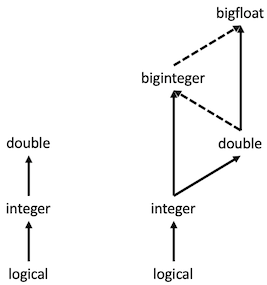# Hierarchy of data types

This vignette follows terminology outlined by the vctrs package. For further information, see help("faq-compatibility-types", package = "vctrs").

There are three numeric types in base R: logical, integer and double. They form a natural hierarchy from the simplest (logical) to the richest (double), with richer types able to accommodate simpler types without losing information.

• integer expands the set of integer values supported by logical.
• double expands the set of integer values supported by integer, and also supports non-integer values.

The bignum package provides two additional numeric types: biginteger and bigfloat. These are type-compatible with the existing numeric types because they extend the set of possible values. However, the hierarchy becomes more complex because lossy casts are now possible.

• biginteger expands the set of integer values supported by double. In fact, it supports any integer value (because biginteger uses arbitrary precision). But it does not support non-integer values.
• bigfloat expands the set of values supported by double (both in precision and range), but does not support the entire range of integers supported by biginteger (because bigfloat uses fixed precision).Summary of numeric type hierarchies in base R and the bignum package. Arrows indicate the direction of richer data types. Dashed lines indicate the potential for lossy casts.

## Type conversion and lossy casts

As discussed above, casting values from one type to another can lose information.

We see an example in base R, when we cast a non-integer or large double to an integer:

# non-integer double
as.integer(1.5)
#>  1
# large double
as.integer(1e10)
#> Warning: NAs introduced by coercion to integer range
#>  NA

For illustrative purposes, we now consider how lossy casts can affect bignum conversions:

library(bignum)

# double -> biginteger
as_biginteger(1.5)
#> Warning: Loss of precision while converting from <double> to <biginteger>.
#> * Locations: 1
#> <biginteger>
#>  1
# biginteger -> double
as.double(biginteger(10)^16L)
#> Warning: Loss of precision while converting from <biginteger> to <double>.
#> * Locations: 1
#>  1e+16
# bigfloat -> double
as.double(bigfloat(1) / 3)
#> Warning: Loss of precision while converting from <bigfloat> to <double>.
#> * Locations: 1
#>  0.3333333
# bigfloat -> biginteger
as_biginteger(bigfloat(1.5))
#> Warning: Loss of precision while converting from <bigfloat> to <biginteger>.
#> * Locations: 1
#> <biginteger>
#>  1
# biginteger -> bigfloat
as_bigfloat(biginteger(10)^51L + 1L)
#> Warning: Loss of precision while converting from <biginteger> to <bigfloat>.
#> * Locations: 1
#> <bigfloat>
#>  1e+51